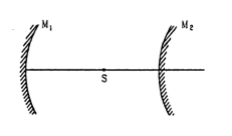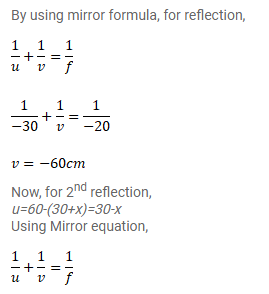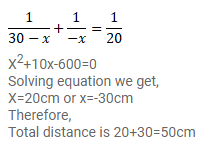# A converging mirror M1, a point source S and a diverging mirror M2Question:

A converging mirror $\mathrm{M}_{1}$, a point source $\mathrm{S}$ and a diverging mirror $\mathrm{M}_{2}$ are arranged as shown in figure The source is placed at a distance of $30 \mathrm{~cm}$ from $\mathrm{M}_{1}$. The focal length of each of the mirror is $20 \mathrm{~cm}$. Consider only the images formed by a maximum of two reflections. It is found that one image is formed on the source itself. (a) Find the distance between the two mirrors. (b) Find the location of the images formed by the single reflection from $\mathrm{M}_{2}$.Solution: